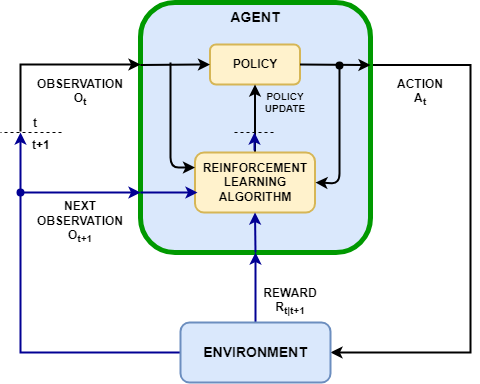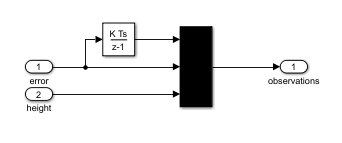Documentation

Create Simulink Environments for Reinforcement Learning

In a reinforcement learning scenario, where you are training an agent to complete task, the environment models the dynamics with which the agent interacts. As shown in the following figure, the environment:

1. Receives actions from the agent

2. Outputs observations in response to the actions

3. Generates a reward measuring how well the action contributes to achieving the taskCreating an environment model includes defining the following:

• Action and observation signals that the agent uses to interact with the environment.

• Reward signal that the agent uses to measure its success. For more information, see Define Reward Signals.

• Environment dynamic behavior.

Action and Observation Signals

When you create an environment object, you must specify the action and observation signals that the agent uses to interact with the environment. You can create both discrete and continuous action spaces. For more information, see rlNumericSpec and rlFiniteSetSpec, respectively.

What signals you select as actions and observations depends on your application. For example, for control system applications, the integrals (and sometimes derivatives) of error signals are often useful observations. Also, for reference-tracking applications, having a time-varying reference signal as an observation is helpful.

When you define your observation signals, ensure that all the system states are observable through the observations. For example, an image observation of a swinging pendulum has position information but does not have enough information to determine the pendulum velocity. In this case, you can specify the pendulum velocity as a separate observation.

Reinforcement Learning Toolbox™ software provides predefined Simulink® environments for which the actions, observations, rewards, and dynamics are already defined. You can use these environments to:

• Learn reinforcement learning concepts

• Gain familiarity with Reinforcement Learning Toolbox software features

• Test your own reinforcement learning agents

To specify your own custom reinforcement learning environment, create a Simulink model with an RL Agent block. In this model, connect the action, observation, and reward signals to the RL Agent block.

For the action and observation signals, you must create specification objects using rlNumericSpec for continuous signals and rlFiniteSetSpec for discrete signals. For bus signals, create specifications using bus2RLSpec.

For the reward signal, construct a scalar signal in the model, and connect this signal to the RL Agent block. For more information, see Define Reward Signals.

After configuring the Simulink model, create an environment object for the model using the rlSimulinkEnv function.

If you have a reference model with an appropriate action input port, observation output port, and scalar reward output port, you can automatically create a Simulink model that includes this reference model and an RL Agent block. For more information, see createIntegratedEnv. This function returns the environment object, action specifications, and observation specifications for the model.

Your environment can include third-party functionality. For more information, see Integrate with Existing Simulation or Environment (Simulink)

Water Tank Environment Model

This example creates a water tank reinforcement learning Simulink® environment that contains an RL Agent block in the place of a controller for the water level in a tank. To simulate this environment, you must create an agent and specify that agent in the RL Agent block. For an example that trains an agent using this environment, see Create Simulink Environment and Train Agent.

mdl = 'rlwatertank';
open_system(mdl)The RL Agent block is connected to the following signals:

• Scalar action output signal

• Vector of observation input signals

• Scalar reward input signal

• Logical input signal for stopping the simulation

Actions and Observations

A reinforcement learning environment receives action signals from the agent and generates observation signals in response to these actions. To create and train an agent, you must create action and observation specification objects.

The action signal for this environment is the flow rate control signal that is sent to the plant. To create a specification object for this continuous action signal, use the rlNumericSpec function.

actionInfo = rlNumericSpec([1 1]);
actionInfo.Name = 'flow';

If the action signal takes one of a discrete set of possible values, create the specification using the rlFiniteSetSpec function.

For this environment, there are three observation signals sent to the agent, specified as a vector signal. The observation vector is ${\left[\begin{array}{ccc}\int \mathit{e}\text{\hspace{0.17em}}\mathrm{dt}& \mathit{e}& \mathit{h}\end{array}\right]}^{\mathit{T}\text{\hspace{0.17em}}}$, where:

• $\mathit{h}$ is the height of the water in the tank

• $\mathit{e}=\mathit{r}-\mathit{h}$, where $\mathit{r}$ is the reference value for the water height

Compute the observation signals in the generate observations subsystem.

open_system([mdl '/generate observations'])Create a three-element vector of observation specifications. Specify a lower bound of 0 for the water height, leaving the other observation signals unbounded.

observationInfo = rlNumericSpec([3 1],...
'LowerLimit',[-inf -inf 0  ]',...
'UpperLimit',[ inf  inf inf]');
observationInfo.Name = 'observations';
observationInfo.Description = 'integrated error, error, and measured height';

If the actions or observations are represented by bus signals, create specifications using the bus2RLSpec function.

Reward Signal

Construct a scalar reward signal. For this example, specify the following reward.

$\mathrm{reward}=10\left(|\mathit{e}|<0.1\right)-1\left(|\mathit{e}|\ge 0.1\right)-100\left(\mathit{h}\le 0||\mathit{h}\ge 20\right)$

The reward is positive when the error is below 0.1 and negative otherwise. Also, there is a large reward penalty when the water height is outside 0 to 20 range.

Construct this reward in the calculate reward subsystem.

open_system([mdl '/calculate reward'])Stop Signal

To terminate training episodes and simulations, specify a logical signal to the isdone input port of the block. For this example, terminate the episode if $\mathit{h}\le 0$ or $\mathit{h}\ge 20$.

Compute this signal in the stop simulation subsystem.

open_system([mdl '/stop simulation'])Create Environment Object

Create an environment object for the Simulink model.

env = rlSimulinkEnv(mdl,[mdl '/RL Agent'],observationInfo,actionInfo);

Reset Function

You can also create a custom reset function that randomizes parameters, variables, or states of the model. In this example, the reset function randomizes the reference signal and the initial water height and sets the corresponding block parameters.

env.ResetFcn = @(in)localResetFcn(in);

Local Function

function in = localResetFcn(in)

% randomize reference signal
blk = sprintf('rlwatertank/Desired \nWater Level');
h = 3*randn + 10;
while h <= 0 || h >= 20
h = 3*randn + 10;
end
in = setBlockParameter(in,blk,'Value',num2str(h));

% randomize initial height
h = 3*randn + 10;
while h <= 0 || h >= 20
h = 3*randn + 10;
end
blk = 'rlwatertank/Water-Tank System/H';
in = setBlockParameter(in,blk,'InitialCondition',num2str(h));

end